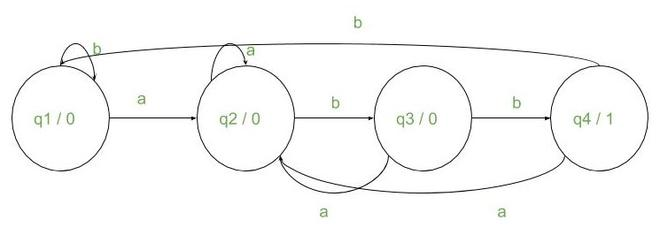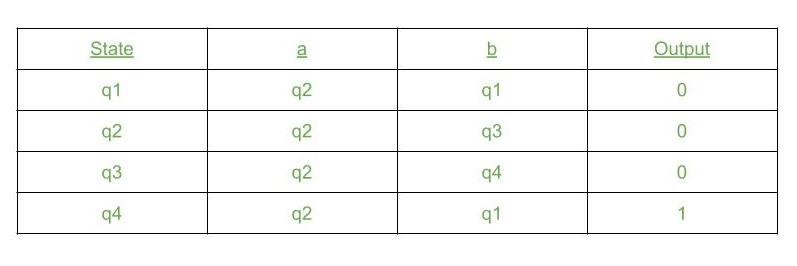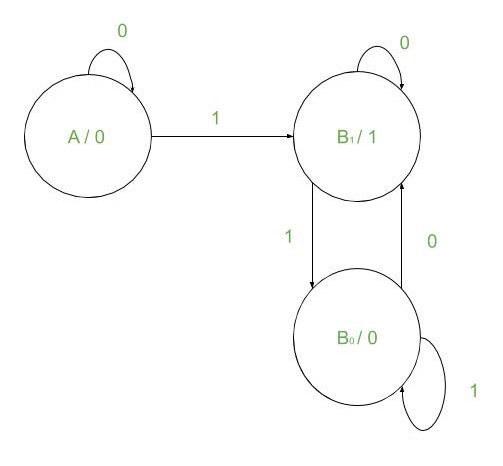Open in App
Not now

# Moore Machines implementation in C++

• Last Updated : 29 Dec, 2022

Moore Machines: A Moore Machine is basically a DFA with an output associated with every state. These machines can be used for a wide variety of tasks such as counting occurrences of a particular substring in a given string, finding 2’s complement of a binary number, etc.

Working of Moore Machine:

• It has an output associated with each state.
• On taking input, it goes to the next state.
• On reaching the next state, it prints the output of the next state.
• This continues until the end of input is reached.

### Some Applications of Moore Machine

Application 1:
Given a string S consisting of a and b, and a substring “abb”, the task is to count the occurrence of the given substring str in the given string S using Moore Machines.

Examples:

Input: S = “babbbbabb”
Output:
00010001
occurrences: 2
Explanation:
The substring “abb” occurs two times in the given string.
Hence, for every substring “abb” a ‘1’ is produced.
The number of 1s is 2.
Input: S = “ab”
Output:
000
occurrences: 0
Explanation:
There is no occurrence of substring “abb” in the given string.
Hence, the number of 1s is 0.

Approach:
The required Moore Machine for this problem is given by:The Transition table for the machine is given below:To implement this, create a structure for mapping input to its next state:

```struct item
{
int value;
State* next;
};```

Then, include this struct as a data member to our class State. The class has three data members:

• Input_1: This is a variable of type item defined above. It maps the first type of input ‘a’ to its next State.
• Input_2: This is also a variable of type item. It maps the second type of input ‘b’ to its next State.
• m_out: This is the output associated with each state of the Moore Machine.

Each object of the class behaves as a state. It takes input and goes to the appropriate next state. To go to the next state, an object pointer can be used. Every object also has an input associated with it.

The following member functions will be used to work on this data:

• Initialize(): This initializes the class object(state) with inputs and corresponding next state(s).
• Transition(): This acts as a transition table for machine. It takes a character from the input string and passes it to the current state which then goes into appropriate next state after producing an output.
• Traverse(): This function takes an input string and character by character passes it into the transition function and return the output string.
• mooreOut(): This function defines the required states(objects) and initializes them to required values. Then passes the input string to the traverse function and receives output string.
• countStr(): This function counts the occurrences of 1 in the output string and returns it.

The next step is to store the current state of the machine while passing string inputs to it. This can be done by using a static Object pointer as a class data member:

Below is the implementation of the above approach:

## C++14

 `// C++ program for the above approach` `#include ``using` `namespace` `std;` `// Define a class named State``class` `State {``private``:``    ``// Item``    ``struct` `item {``        ``char` `value;``        ``State* next;``    ``};` `    ``// Three states``    ``item Input1;``    ``item Input2;``    ``char` `m_out;` `public``:``    ``// Constructor``    ``State()``        ``: Input1{ ``' '``, nullptr },``          ``Input2{ ``' '``, nullptr },``          ``m_out{ ``' '` `}``    ``{``    ``}` `    ``// Member functions``    ``static` `State* m_ptr;``    ``void` `Initialize(item input1,``                    ``item input2,``                    ``char` `out);``    ``static` `char` `Transition(``char` `x);``    ``static` `string Traverse(string& str,``                           ``int` `n);``};` `// Global object pointer points to``// current state``State* State::m_ptr{ nullptr };` `// Function that initializes the states``// with appropriate values``void` `State::Initialize(item input1,``                       ``item input2,``                       ``char` `out)``{``    ``Input1 = input1;``    ``Input2 = input2;``    ``m_out = out;``}` `// Transition function that takes each``// character of string``char` `State::Transition(``char` `x)``{``    ``char` `ch{};` `    ``// Prints the output``    ``if` `((*m_ptr).Input1.value == x) {` `        ``// Output the current state``        ``cout << (*m_ptr).m_out;``        ``ch = (*m_ptr).m_out;` `        ``// Next input state``        ``m_ptr = (*m_ptr).Input1.next;``    ``}``    ``else` `{` `        ``// Output the current state``        ``cout << (*m_ptr).m_out;``        ``ch = (*m_ptr).m_out;` `        ``// Next input state``        ``m_ptr = (*m_ptr).Input2.next;``    ``}` `    ``// Return ch``    ``return` `ch;``}` `// Takes the whole string and pass``// it through machine``string State::Traverse(string& str,``                       ``int` `n)``{``    ``string str1{};` `    ``// Add all the transition state to``    ``// the string str1``    ``for` `(``int` `i = 0; i < n; i++)``        ``str1 += Transition(str[i]);` `    ``// Append output``    ``str1 += (*m_ptr).m_out;``    ``cout << (*m_ptr).m_out << endl;` `    ``// Return str1``    ``return` `str1;``}` `// Function that create states and``// produce output``string mooreOut(string str, ``int` `n)``{``    ``State q1, q2, q3, q4;` `    ``// Initializing the states``    ``q1.Initialize({ ``'a'``, &q2 },``                  ``{ ``'b'``, &q1 }, ``'0'``);``    ``q2.Initialize({ ``'a'``, &q2 },``                  ``{ ``'b'``, &q3 }, ``'0'``);``    ``q3.Initialize({ ``'a'``, &q2 },``                  ``{ ``'b'``, &q4 }, ``'0'``);``    ``q4.Initialize({ ``'a'``, &q2 },``                  ``{ ``'b'``, &q1 }, ``'1'``);``    ``State::m_ptr = &q1;` `    ``// Traverse the string str1``    ``string str1{ State::Traverse(str, n) };``    ``return` `str1;``}` `// Function that counts the occurrences``// of 1 in the output string``int` `countStr(string& str, ``int` `n)``{``    ``int` `count{};` `    ``// Count the 1s in str``    ``for` `(``int` `i = 0; i < n; i++) {``        ``if` `(str[i] == ``'1'``)``            ``count++;``    ``}` `    ``// Return count``    ``return` `count;``}` `// Driver Code``int` `main()``{` `    ``// Given string``    ``string str{ ``"babbabbabbb"` `};` `    ``int` `n{ ``static_cast``<``int``>(str.length()) };` `    ``// Function Call``    ``string str1{ mooreOut(str, n) };``    ``int` `n1{ ``static_cast``<``int``>(str.length()) };` `    ``// Print the count of substring``    ``cout << ``"abb occurs "` `<< countStr(str1, n1)``         ``<< ``" times\n"``;``    ``return` `0;``}`

Output:

```000010010010
abb occurs 3 times```

Time Complexity: O(N)
Auxiliary Space: O(N)
Application 2:
Given a binary string str, the task is to find the 2s complement of the given string str.

Input: str = “111010000”
Output: 000110000
Input: str = “111”
Output: 001

Approach: The idea is to start from the rightmost bit and pass it into the machine giving the output. Pass the whole string like this, going from right to left. The following observations can be observed:
For Example: The given string is “111010000”. Now, 2s complement is given by 1s complement of(str) + 1. Therefore,

```str =           "111010000"
1s complement = "000101111"
+        1
---------------------------
2s complement =  000110000```

Here we can observe that from the rightmost bit the bits are copied the same way until 1 appears. After that all the bits are reversed.
Therefore, the idea is to define a Moore Machine to start to take input from the rightmost side. As long as the bit is 0 it gives the same output(0). When 1 is encountered then it gives 1 for that. After this, for any bit taken as input its inverse is given as output.

The Moore Machine for this problem is given below:To implement this first, map the transition table for this machine. Three states are needed i.e. three objects of class State defined at the beginning:

• State1 => Initialize( {‘0’, &State1}, {‘1’, &State2}, ‘0’)
• State2 => Initialize( {‘0’, &State2}, {‘1’, &State3}, ‘1’)
• State3 => Initialize( {‘0’, &State2}, {‘1’, &State3}, ‘0’)

After initialization, a Transition function is needed like the one defined above. It takes an input and for that prints output of the current state and goes to the next state by mapping input to the next state using the transition table defined above. Then to traverse the string, the transition begins from the rightmost bit and goes till the leftmost bit.

Below is the implementation of the above approach:

## C++

 `// C++ program for the above approach` `#include ``using` `namespace` `std;` `// Define a class named State``class` `State {``private``:``    ``struct` `item {``        ``char` `value;``        ``State* next;``    ``};``    ``item Input1;``    ``item Input2;``    ``char` `m_out;` `public``:``    ``// Constructors``    ``State()``        ``: Input1{ ``' '``, nullptr },``          ``Input2{ ``' '``, nullptr },``          ``m_out{ ``' '` `}``    ``{``    ``}``    ``static` `State* m_ptr;` `    ``// Member Functions``    ``void` `Initialize(item input1,``                    ``item input2,``                    ``char` `out);``    ``static` `char` `Transition(``char` `x);``    ``static` `string Traverse(string& str,``                           ``int` `n);``};` `// Global object pointer points to``// current state``State* State::m_ptr{ nullptr };` `// Function that initializes the states``// with appropriate values``void` `State::Initialize(item input1,``                       ``item input2,``                       ``char` `out)``{``    ``Input1 = input1;``    ``Input2 = input2;``    ``m_out = out;``}` `// Transition function takes each``// character of string``char` `State::Transition(``char` `x)``{``    ``char` `ch{};` `    ``// Prints the output``    ``if` `((*m_ptr).Input1.value == x) {` `        ``// Output the current state``        ``cout << (*m_ptr).m_out;``        ``ch = (*m_ptr).m_out;` `        ``// Next input state``        ``m_ptr = (*m_ptr).Input1.next;``    ``}``    ``else` `{` `        ``// Output the current state``        ``cout << (*m_ptr).m_out;``        ``ch = (*m_ptr).m_out;` `        ``// Next input state``        ``m_ptr = (*m_ptr).Input2.next;``    ``}` `    ``// Return ch``    ``return` `ch;``}` `// Takes the whole string and passes``// through machine``string State::Traverse(string& str, ``int` `n)``{``    ``string str1{};` `    ``// Add all the transition state to``    ``// the string str1``    ``for` `(``int` `i = n - 1; i >= 0; i--) {``        ``str1 += Transition(str[i]);``    ``}` `    ``// To read the characters from end``    ``// therefore we need to reverse``    ``reverse(str1.begin(), str1.end());` `    ``return` `str1;``}` `// Function to create states and``// produce output``string mooreOut(string str, ``int` `n)``{``    ``State q1, q2, q3;` `    ``// Initializing the states``    ``q1.Initialize({ ``'0'``, &q1 },``                  ``{ ``'1'``, &q2 }, ``'0'``);``    ``q2.Initialize({ ``'0'``, &q2 },``                  ``{ ``'1'``, &q3 }, ``'1'``);``    ``q3.Initialize({ ``'0'``, &q2 },``                  ``{ ``'1'``, &q3 }, ``'0'``);``    ``State::m_ptr = &q1;``    ``return` `State::Traverse(str, n);``}` `// Driver Code``int` `main()``{``    ``// Given string``    ``string str{ ``"111010000"` `};``    ``int` `n{ ``static_cast``<``int``>(str.length()) };` `    ``// Function Call``    ``string str1{ mooreOut(str, n) };` `    ``// Print the output``    ``cout << ``"2's complement: "` `<< str1;``    ``return` `0;``}`

Output:

`2's complement: 000110000`

Time Complexity: O(N), where N is the length of the given binary string.
Auxiliary Space: O(N)

My Personal Notes arrow_drop_up